We require the following R packages.

# Install necessary packages
list_packages <- c("forecast", "readxl", "stargazer", "fpp",
"fpp2", "scales", "quantmod", "urca",
"vars", "tseries", "ggplot2", "dplyr")
new_packages <- list_packages[!(list_packages %in% installed.packages()[,"Package"])]
if(length(new_packages)) install.packages(new_packages)

lapply(list_packages, require, character.only = TRUE)

# 1 Model Identification

Given time series data, we need to identify the form of the model first, i.e., AR, MA, ARMA, or ARIMA? The order (p,d,q)?

The main tool is sample ACF $$\hat{\rho}_k$$ and sample PACF $$\hat{\phi}_{kk}$$.

Model ACF, $$\rho_k$$ PACF, $$\phi_{kk}$$
AR(p) exponentially decay cut off at lag $$p$$
MA(q) cut off at lag $$q$$ exponentially decay
ARMA(p,q) exponentially decay after lag $$q$$ exponentially decay
ARIMA(p,d,q) slowly decrease exponentially decay

Model identification requires a good understanding the process AR, MA, ARMA, ARIMA, for example, their ACFs and PACFs.

In practice, these ACF and PACF are unknown. For given time series data, ACF and PACF have to be estimated.

In model identification, our goal is to match patterns in the sample ACF and PACF with the known patterns of ACF and PACF of a specific model.

To get sample ACF and PACF, we use:

In R, we use

acf()
pacf()

In SAS, we use

IDENTIFY VAR=Z NLAG =50

Examples

Simulated data:

n=200
ts1=arima.sim(n = n, list(order = c(1,0,0), ar = c(0.5)), sd = 1)
par(mfrow=c(1,3))
plot.ts(ts1)
acf(ts1,ylim=c(-1,1),lag.max=10)
pacf(ts1,ylim=c(-1,1),lag.max=10)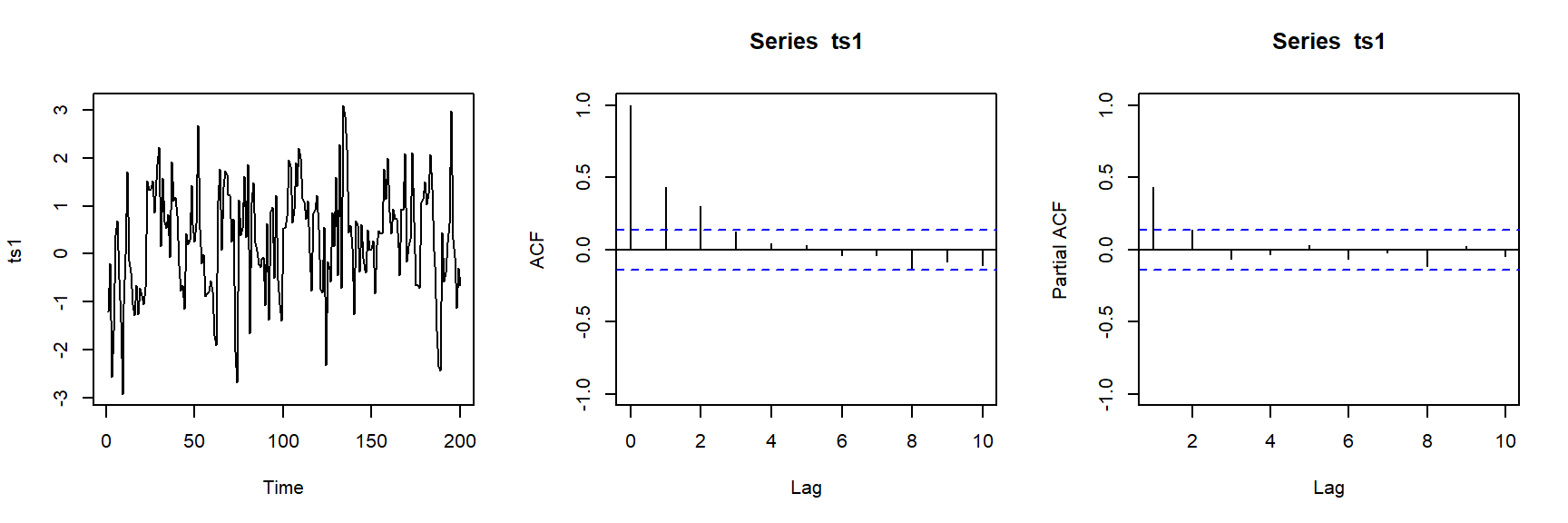n=20000
ts1=arima.sim(n = n, list(order = c(1,0,0), ar = c(0.5)), sd = 1)
par(mfrow=c(1,3))
plot.ts(ts1)
acf(ts1,ylim=c(-1,1),lag.max=10)
pacf(ts1,ylim=c(-1,1),lag.max=10)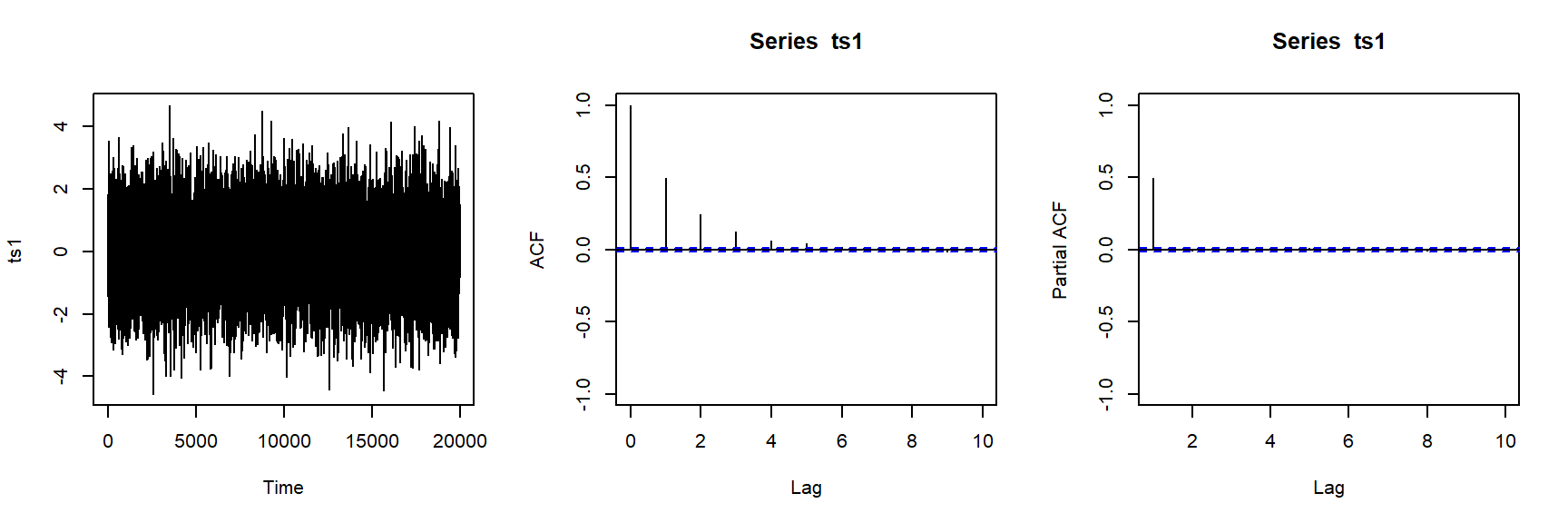# 2 Cases

Case 1: Business Inventories data fWe analyzed the quarterly change in bupiness inventories, stated at annual rates in billions of dollars. We examine 60 observations covering the period from the first quarter of 1955 through the fourth quarter of 1969. The data is seasonally adjusted.

case1=as.ts(scan("case1.txt"))
case1
## Time Series:
## Start = 1
## End = 60
## Frequency = 1
##    4.4  5.8  6.7  7.1  5.7  4.1  4.6  4.3  2.0  2.2  3.6 -2.2 -5.1 -4.9  0.1
##   4.1  3.8  9.9  0.0  6.5 10.8  4.1  2.7 -2.9 -2.9  1.5  5.7  5.0  7.9  6.8
##   7.1  4.1  5.5  5.1  8.0  5.6  4.5  6.1  6.7  6.1 10.6  8.6 11.6  7.6 10.9
##  14.6 14.5 17.4 11.7  5.8 11.5 11.7  5.0 10.0  8.9  7.1  8.3 10.2 13.3  6.2
par(mfrow=c(1,3))
plot(case1)
acf(case1)
pacf(case1)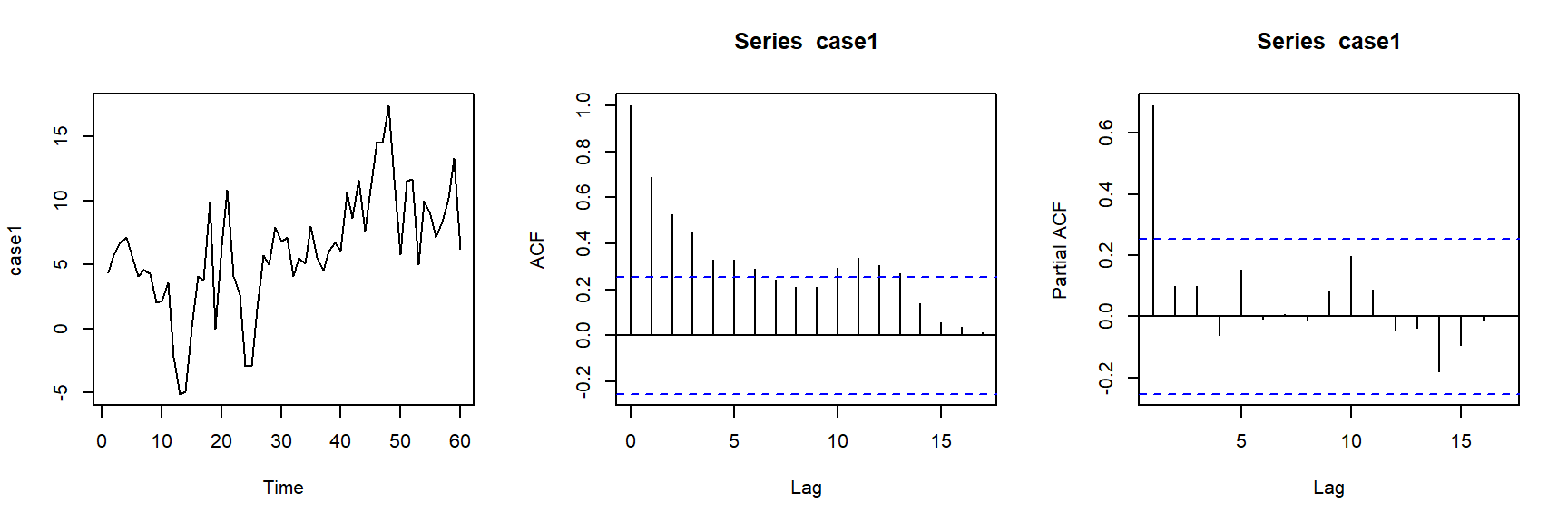fit <- arima(case1,order=c(1,0,0))
fit
##
## Call:
## arima(x = case1, order = c(1, 0, 0))
##
## Coefficients:
##          ar1  intercept
##       0.6803     6.0423
## s.e.  0.0914     1.2870
##
## sigma^2 estimated as 10.88:  log likelihood = -157.04,  aic = 320.08
par(mfrow=c(1,2))
plot(forecast(fit,h=30))
fitauto <- auto.arima(case1)
fitauto
## Series: case1
## ARIMA(1,1,1)
##
## Coefficients:
##          ar1      ma1
##       0.5017  -0.8767
## s.e.  0.1643   0.0918
##
## sigma^2 estimated as 11.34:  log likelihood=-154.63
## AIC=315.26   AICc=315.7   BIC=321.5
plot(forecast(fitauto,h=30))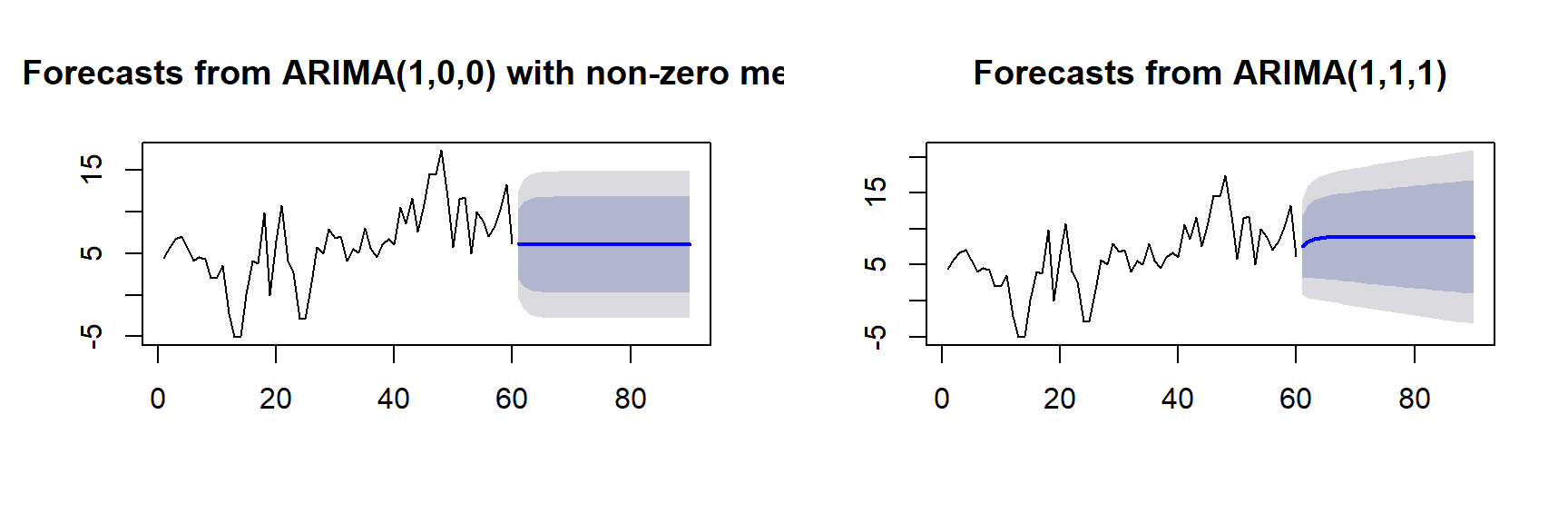Case 2: Saving Rate dataThe saving rate is personal saving as a percent of disposable personal income. Some economists believe shifts in this rate contribute to business fluctuations. For example, when people save more of their income they spend less for goods and services. This reduction in total demand for output may cause national production to fall and unemployment to rise.

We analyze 100 quarterly observations of the US saving rate for the years 1955-1979. The data is seasonally adjusted.

case2=as.ts(scan("case2.txt"))
case2
## Time Series:
## Start = 1
## End = 100
## Frequency = 1
##    4.9 5.2 5.7 5.7 6.2 6.7 6.9 7.1 6.6 7.0 6.9 6.4 6.6 6.4 7.0 7.3 6.0 6.3
##   4.8 5.3 5.4 4.7 4.9 4.4 5.1 5.3 6.0 5.9 5.9 5.6 5.3 4.5 4.7 4.6 4.3 5.0
##   5.2 6.2 5.8 6.7 5.7 6.1 7.2 6.5 6.1 6.3 6.4 7.0 7.6 7.2 7.5 7.8 7.2 7.5
##   5.6 5.7 4.9 5.1 6.2 6.0 6.1 7.5 7.8 8.0 8.0 8.1 7.6 7.1 6.6 5.6 5.9 6.6
##   6.8 7.8 7.9 8.7 7.7 7.3 6.7 7.5 6.4 9.7 7.5 7.1 6.4 6.0 5.7 5.0 4.2 5.1
##   5.4 5.1 5.3 5.0 4.8 4.7 5.0 5.4 4.3 3.5
par(mfrow=c(1,3))
plot(case2)
acf(case2)
pacf(case2)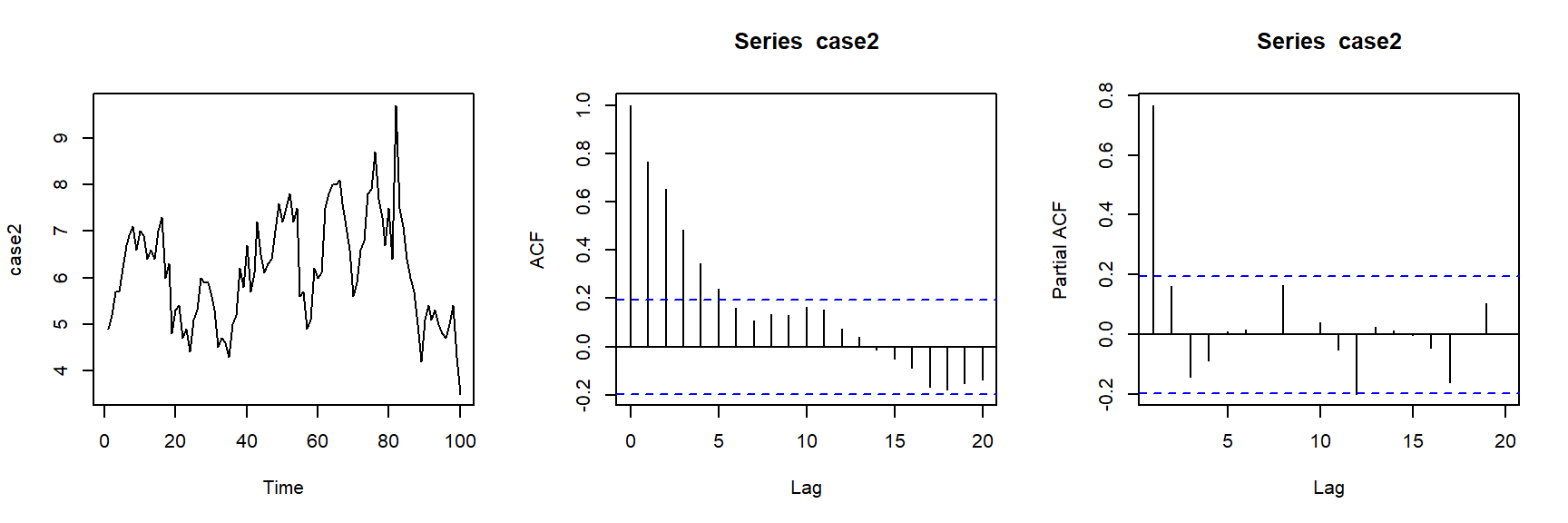Case 3: Coal Production data The data in this case is monthly bituminous coal production in the US from January 1952 through December 1959, a total of 96 observations. The data is seasonally adjusted.

case3=as.ts(scan("case3.txt"))
case3
## Time Series:
## Start = 1
## End = 96
## Frequency = 1
##   47730 46704 41535 41319 36962 32558 31995 32993 44834 29883 39611 40099
##  38051 36927 37272 39457 38097 40226 43589 39088 39409 37226 34421 34975
##  32710 31885 32106 30029 29501 31620 34205 32153 32764 33230 35636 35550
##  34529 37498 37229 36021 38281 36676 44541 40850 38404 37575 41476 42267
##  43062 45036 43769 42298 44412 40498 37830 42294 38330 43554 42579 36911
##  42541 42430 43465 44468 43597 40774 42573 41635 39030 41572 37027 34732
##  36817 34295 33218 32034 31417 35719 30001 33096 35196 36550 33463 37195
##  34748 36461 35754 36943 35854 37912 30095 28931 31020 31746 34613 37901
par(mfrow=c(1,3))
plot(case3)
acf(case3)
pacf(case3)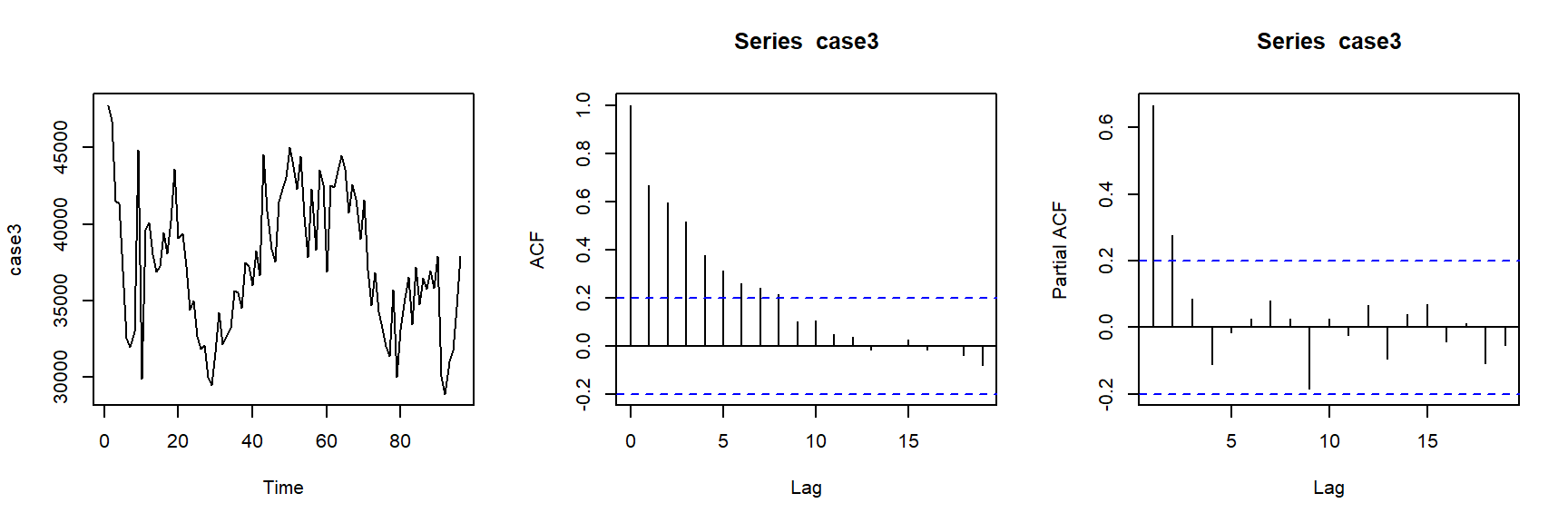Case 4: Housing Permits data We develop a model to forecast the index of new private housing units authorized by local building permits. These are quarterly seasonally adjusted data covering the years 1947-1967.

case4=as.ts(scan("case4.txt"))
case4
## Time Series:
## Start = 1
## End = 84
## Frequency = 1
##    83.3  83.2 105.3 117.7 104.6 108.8  93.9  86.1  83.0 102.4 119.6 141.4
##  158.6 161.3 158.2 136.1 121.9  97.7 103.3  92.7 106.8 102.1 110.3 114.1
##  109.1 105.4  97.6 100.7 102.7 110.9 120.2 131.3 138.9 130.9 123.1 110.8
##  108.8 103.8  97.0  93.2  89.7  89.9  90.2  89.6  85.8  96.9 112.7 122.7
##  119.8 117.4 111.9 104.7  98.3  94.9  93.3  90.9  91.9  97.2 104.7 107.7
##  108.2 110.7 113.1 114.6 112.2 120.2 122.1 126.6 122.3 115.9 116.9 110.1
##  110.4 108.9 112.1 117.6 112.2  96.0  78.0  66.9  83.5  95.8 107.7 113.7
par(mfrow=c(1,3))
plot(case4)
acf(case4)
pacf(case4)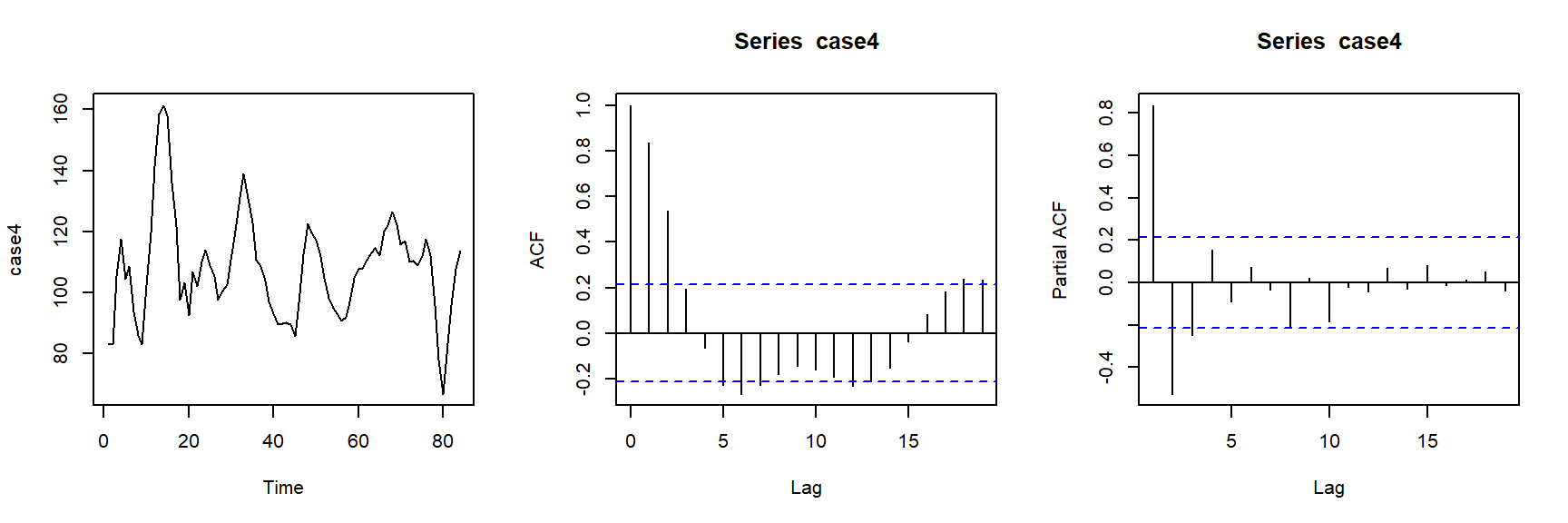Case 5: Rail Freight data We model the quarterly freight volume carried by Class I railroads in the US measured in billions of ton-miles. The data cover the period 1965-1978, a total of 56 observations. The data is seasonally adjusted.

case5=as.ts(scan("case5.txt"))
case5
## Time Series:
## Start = 1
## End = 56
## Frequency = 1
##   166.8 172.8 178.3 180.3 182.6 184.2 188.9 184.4 181.7 178.5 177.6 181.0
##  186.5 185.7 186.4 186.3 189.3 190.6 191.7 196.1 189.3 192.6 192.1 189.4
##  189.7 191.9 182.0 175.7 192.0 192.8 193.3 200.2 208.8 211.4 214.4 216.3
##  221.8 217.1 214.0 202.4 191.7 183.9 185.2 194.5 195.8 198.0 200.9 199.0
##  200.6 209.5 208.4 206.7 193.3 197.3 213.7 225.1
par(mfrow=c(1,3))
plot(case5)
acf(case5)
pacf(case5)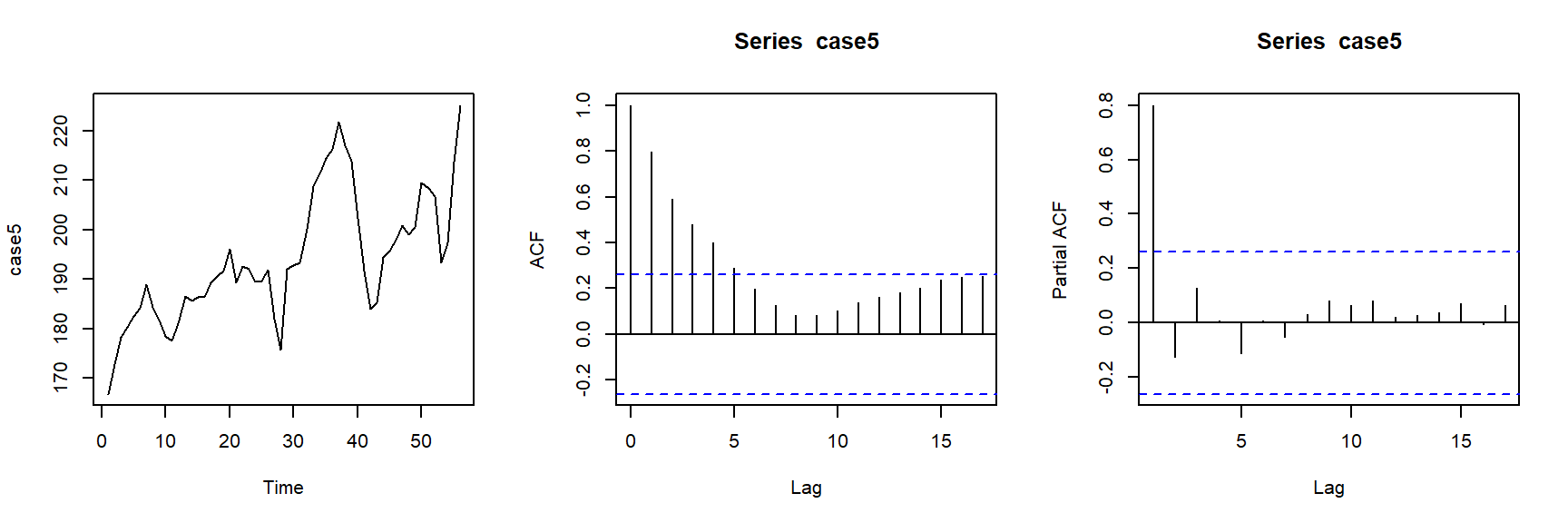Case 6: AT&T Stock Price data The data is the weekly closing price of American Telephone and Telegraph (AT&T) common shares for the year 1979. The observations were taken from various issues of the Wall Street Journal, with only 52 observations.

case6=as.ts(scan("case6.txt"))
case6
## Time Series:
## Start = 1
## End = 52
## Frequency = 1
##   61.000 61.625 61.000 64.000 63.750 63.375 63.875 61.875 61.500 61.625
##  62.125 61.625 61.000 61.875 61.625 59.625 58.750 58.750 58.250 58.500
##  57.750 57.125 57.750 58.875 58.000 57.875 58.000 57.125 57.250 57.375
##  57.125 57.500 58.375 58.125 56.625 56.250 56.250 55.125 55.000 55.125
##  53.000 52.375 52.875 53.500 53.375 53.375 53.500 53.750 54.000 53.125
##  51.875 52.250
par(mfrow=c(1,3))
plot(case6)
acf(case6)
pacf(case6)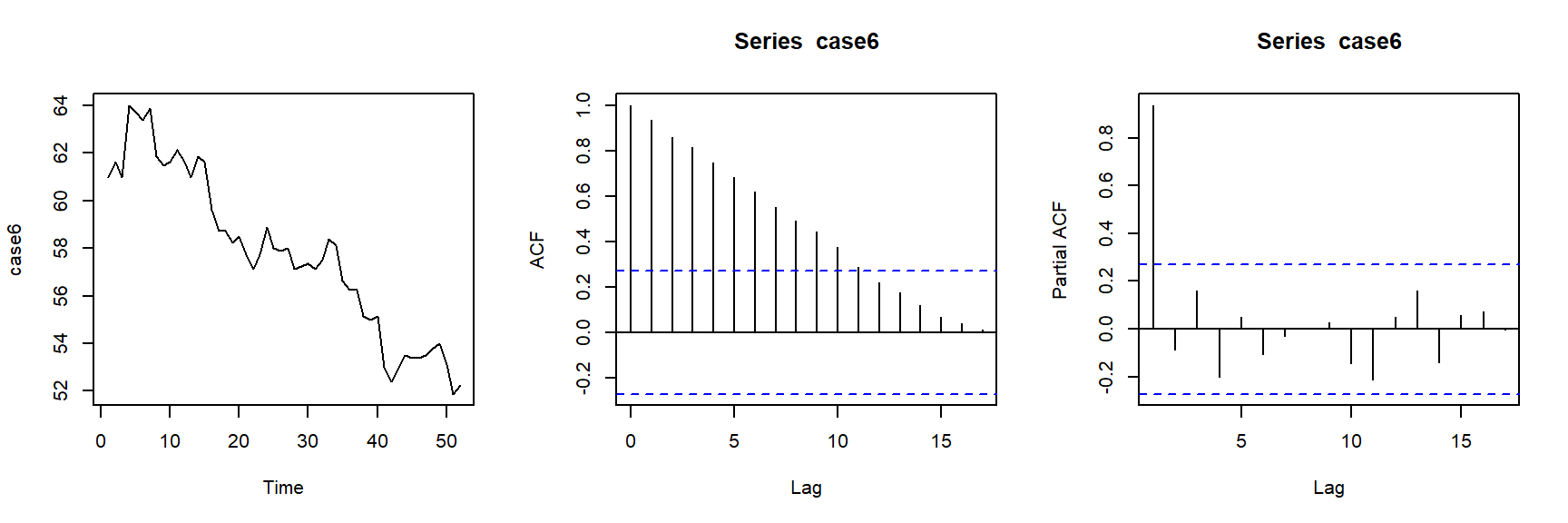Case 7: Real-Estate Loan data We analyze the monthly volume of commercial bank real-estate loans in billions of dollars, from January 1973 to October 1978, a total of 70 observations. The data is derived from reports to the Federal Reserve System from large commercial banks.

case7=as.ts(scan("case7.txt"))
case7
## Time Series:
## Start = 1
## End = 70
## Frequency = 1
##   46.5 47.0 47.5 48.3 49.1 50.1 51.1 52.0 53.2 53.9 54.5 55.2 55.6 55.7 56.1
##  56.8 57.5 58.3 58.9 59.4 59.8 60.0 60.0 60.3 60.1 59.7 59.5 59.4 59.3 59.2
##  59.1 59.0 59.3 59.5 59.5 59.5 59.7 59.7 60.5 60.7 61.3 61.4 61.8 62.4 62.4
##  62.9 63.2 63.4 63.9 64.5 65.0 65.4 66.3 67.7 69.0 70.0 71.4 72.5 73.4 74.6
##  75.2 75.9 76.8 77.9 79.2 80.5 82.6 84.4 85.9 87.6
par(mfrow=c(1,3))
plot(case7)
acf(case7)
pacf(case7)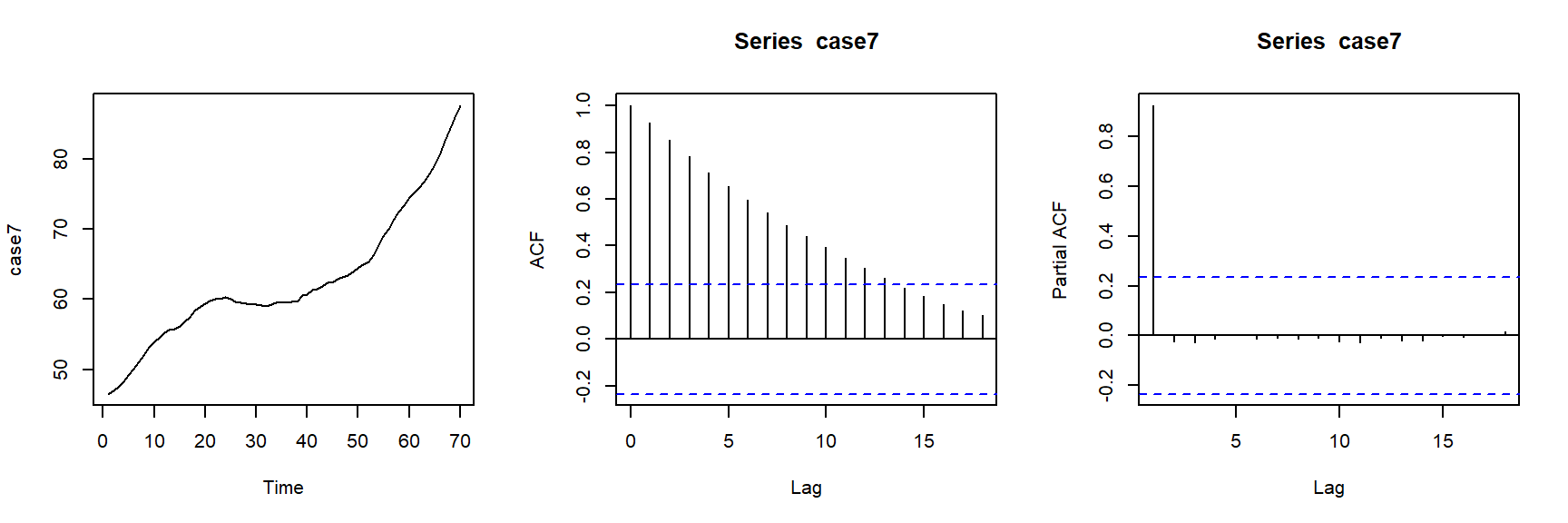Case 9: Air-Carrier Freight data We study the volume of freight, measured in ton-miles, hauled by air carries in the US. There are 120 monthly observations covering the years 1969-1978.

case9=as.ts(scan("case9.txt"))
case9
## Time Series:
## Start = 1
## End = 120
## Frequency = 1
##    1299 1148 1345 1363 1374 1533 1592 1687 1384 1388 1295 1489 1403 1243 1466
##   1434 1520 1689 1763 1834 1494 1439 1327 1554 1405 1252 1424 1517 1483 1605
##   1775 1840 1573 1617 1485 1710 1563 1439 1669 1651 1654 1847 1931 2034 1705
##   1725 1687 1842 1696 1534 1814 1796 1822 2008 2088 2230 1843 1848 1736 1826
##   1766 1636 1921 1882 1910 2034 2047 2195 1765 1818 1634 1818 1698 1520 1820
##   1689 1775 1968 2110 2241 1803 1899 1762 1901 1839 1727 1954 1991 1988 2146
##   2301 2338 1947 1990 1832 2066 1952 1747 2098 2057 2060 2240 2425 2515 2128
##  2255 2116 2315 2143 1948 1460 2344 2363 2630 2811 2972 2515 2536 2414 2545
par(mfrow=c(1,3))
plot(case9)
acf(case9)
pacf(case9)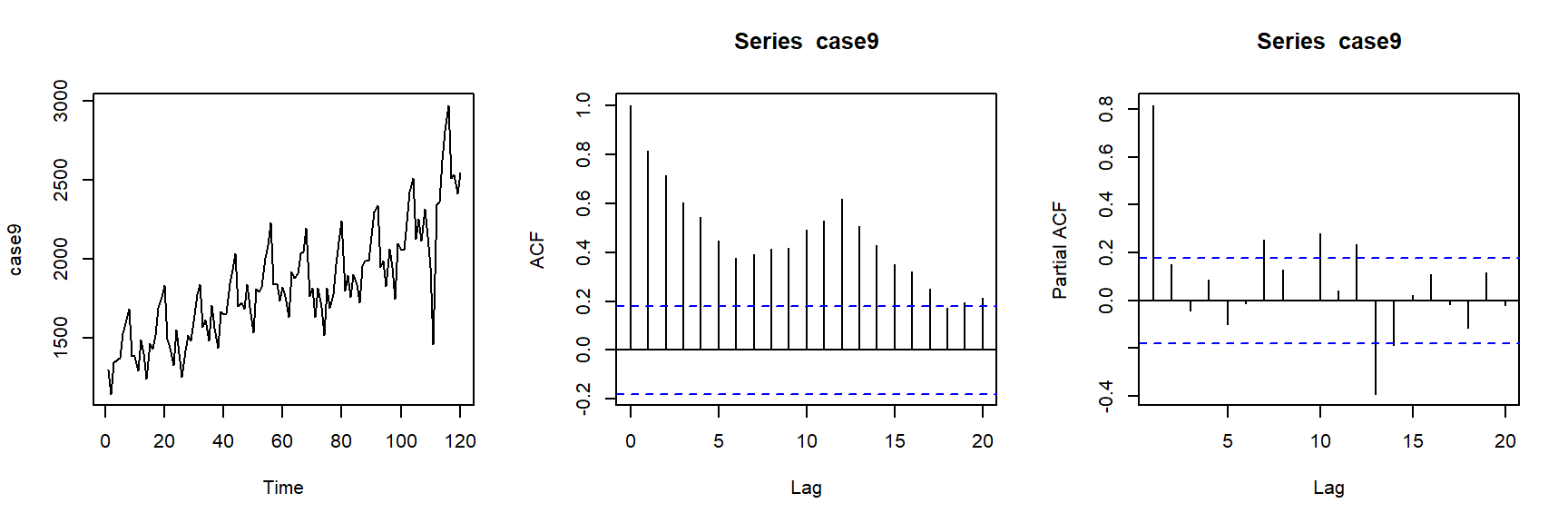Case 10: Profit Margin data The data is the after-tax profits, measured in cents per dollar of sales, for all US manufacturing corporations. The data covers the period 1953-1972, a total of 80 observations.

case10=as.ts(scan("case10.txt"))
case10
## Time Series:
## Start = 1
## End = 80
## Frequency = 1
##   4.4 4.3 4.4 4.0 4.3 4.6 4.5 4.7 5.2 5.4 5.5 5.6 5.4 5.4 5.0 5.1 5.3 4.9 4.7
##  4.3 3.6 3.7 4.4 4.8 5.0 5.3 4.6 4.4 5.0 4.4 4.3 3.9 3.8 4.2 4.4 4.7 4.6 4.4
##  4.5 4.7 4.4 4.7 4.7 5.0 5.1 5.2 5.3 5.3 5.6 5.5 5.6 5.6 5.8 5.7 5.6 5.4 5.0
##  5.0 4.9 5.1 5.1 5.0 5.1 5.1 5.1 4.9 4.8 4.5 4.1 4.2 4.0 3.6 4.0 4.2 4.2 4.1
##  4.2 4.2 4.3 4.5
par(mfrow=c(1,3))
plot(case10)
acf(case10)
pacf(case10)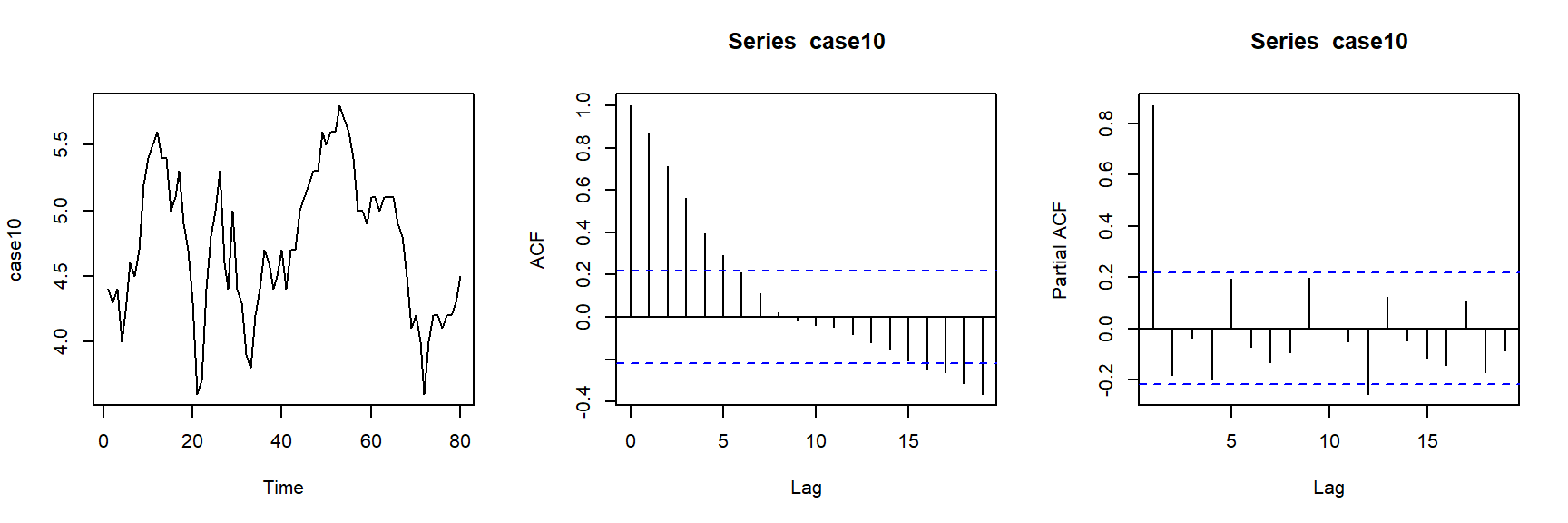Case 13: Cigar Consumption data The data represents monthly cigar consumption (withdrawals from stock) for the years 1969-1976.

case13=as.ts(scan("case13.txt"))
case13
## Time Series:
## Start = 1
## End = 96
## Frequency = 1
##   484 498 537 552 597 576 544 621 604 719 599 414 502 494 527 544 631 557 540
##  588 593 653 582 495 510 506 557 559 571 564 497 552 559 597 616 418 452 460
##  541 460 592 475 442 563 482 562 520 346 466 403 465 485 507 483 403 506 442
##  576 480 339 418 380 405 452 403 379 399 464 443 533 416 314 351 354 372 394
##  397 417 347 371 389 448 349 286 317 288 364 337 342 377 315 356 354 388 340
##  264
par(mfrow=c(1,3))
plot(case13)
acf(case13)
pacf(case13)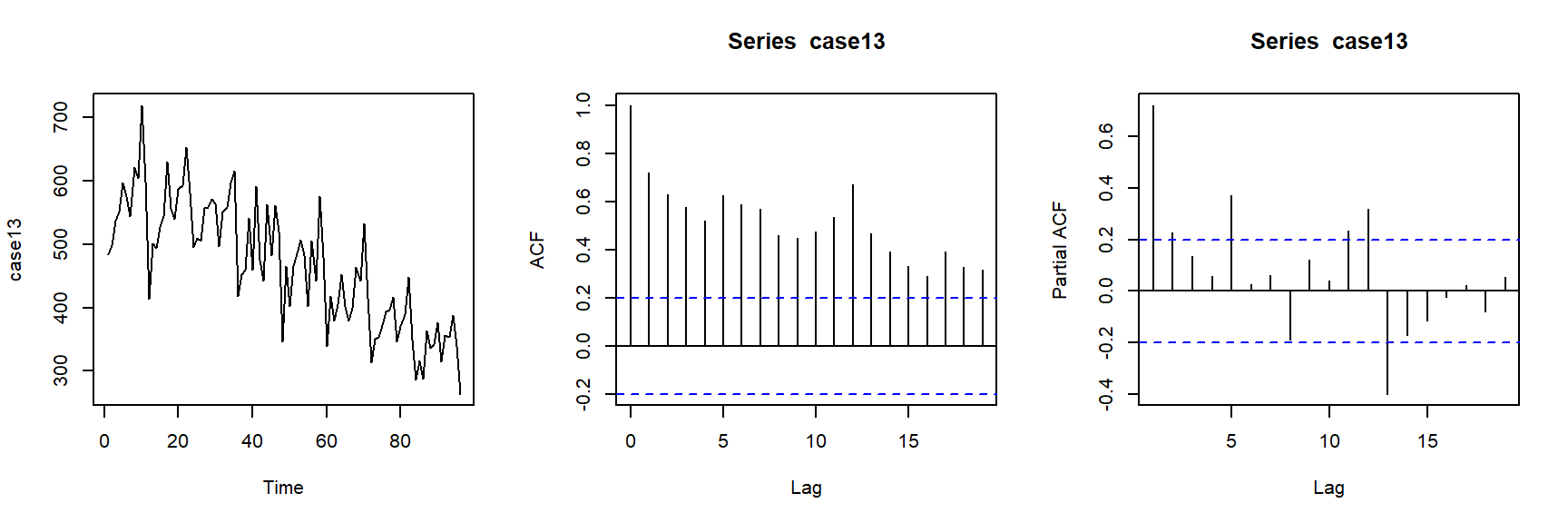Case IMA: Refrigerator daca

d=scan("case_refrigerator.txt")
case_ref=as.ts(d[seq(2,length(d),2)])
case_ref
## Time Series:
## Start = 1
## End = 52
## Frequency = 1
##   390 323 371 326 358 538 533 458 414 489 306 654 458 507 362 367 306 223 281
##  317 238 286 306 307 275 284 298 318 340 497 349 380 379 526 272 401 553 527
##  485 722 474 510 760 515 560 751 842 818 746 672 854 692
par(mfrow=c(1,3))
plot(case_ref)
acf(case_ref)
pacf(case_ref)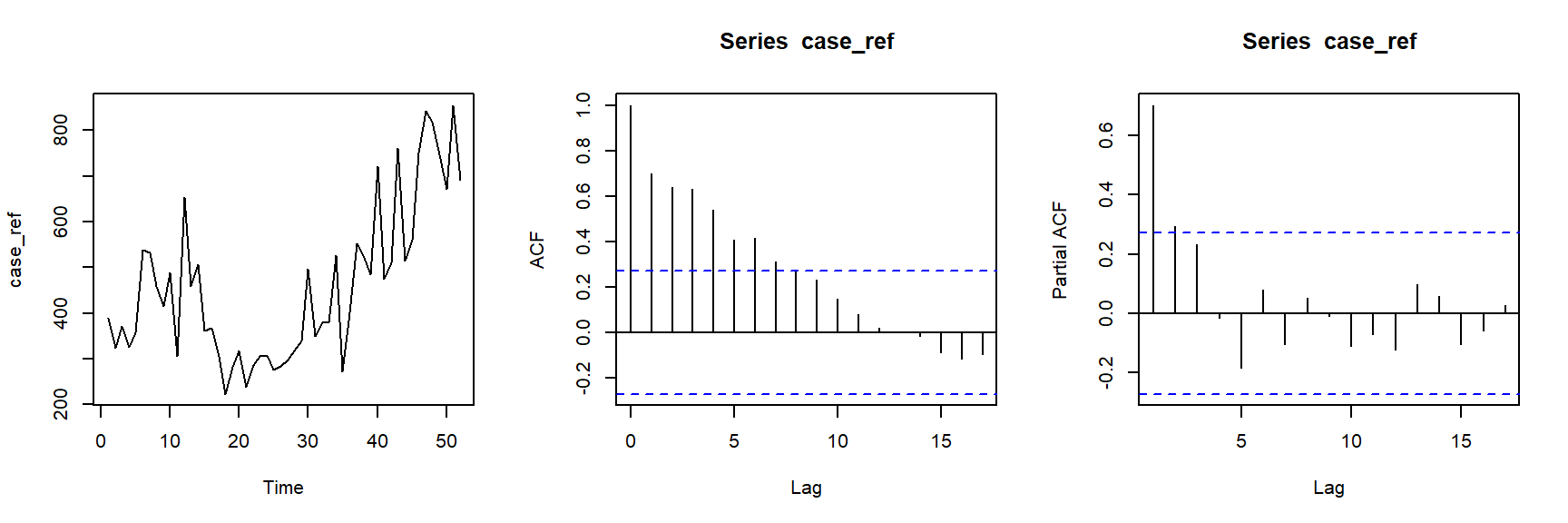Case WWWusage: the numbers of users connected to the Internet through a server every minute.

par(mfrow=c(1,3))
plot.ts(WWWusage)
acf(WWWusage)
pacf(WWWusage)